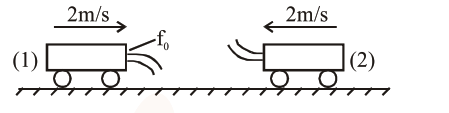# Two cars are approaching`
Question:

Two cars are approaching each other at an equal speed of $7.2 \mathrm{~km} / \mathrm{hr}$. When they see each other, both blow horns having frequency of $676 \mathrm{~Hz}$. The beat frequency heard by each driver will be ____________$\mathrm{Hz}$. [Velocity of sound in air is 340 $\mathrm{m} / \mathrm{s} .]$

Solution:Frequency of sound heard by car- 1 , which comes by reflection from car- 2

$\mathrm{f}_{1}=\mathrm{f}_{0}\left(\frac{340+2}{340-2}\right)\left(\frac{340+2}{340-2}\right)$

$=\mathrm{f}_{0}\left(\frac{342}{338}\right)^{2}$

Frequency of sound coming directly from car-2

$\mathrm{f}_{2}=\mathrm{f}_{0}\left(\frac{340+2}{340-2}\right)$

$\therefore \mathrm{f}_{1}-\mathrm{f}_{2}=\mathrm{f}_{0}\left(\frac{342}{338}\right)\left(\frac{342}{338}-1\right)=8.09 \simeq 8$